## Example Questions

### Example Question #2 : How To Find The Length Of The Diagonal Of A Hexagon

How many diagonals are there in a regular hexagon?Explanation:

A diagonal is a line segment joining two non-adjacent vertices of a polygon.  A regular hexagon has six sides and six vertices.  One vertex has three diagonals, so a hexagon would have three diagonals times six vertices, or 18 diagonals.  Divide this number by 2 to account for duplicate diagonals between two vertices. The formula for the number of vertices in a polygon is: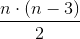where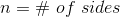.

### Example Question #3 : How To Find The Length Of The Diagonal Of A Hexagon

How many diagonals are there in a regular hexagon?

10

9

3

6

18

9

Explanation:

A diagonal connects two non-consecutive vertices of a polygon.  A hexagon has six sides.  There are 3 diagonals from a single vertex, and there are 6 vertices on a hexagon, which suggests there would be 18 diagonals in a hexagon.  However, we must divide by two as half of the diagonals are common to the same vertices. Thus there are 9 unique diagonals in a hexagon. The formula for the number of diagonals of a polygon is:where n = the number of sides in the polygon.

Thus a pentagon thas 5 diagonals.  An octagon has 20 diagonals.

### Example Question #15 : Geometry

Hexagon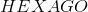is a regular hexagon with sides of length 10.is the midpoint of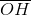. To the nearest tenth, give the length of the segment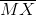.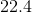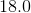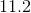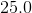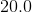Explanation:

Below is the referenced hexagon, with some additional segments constructed.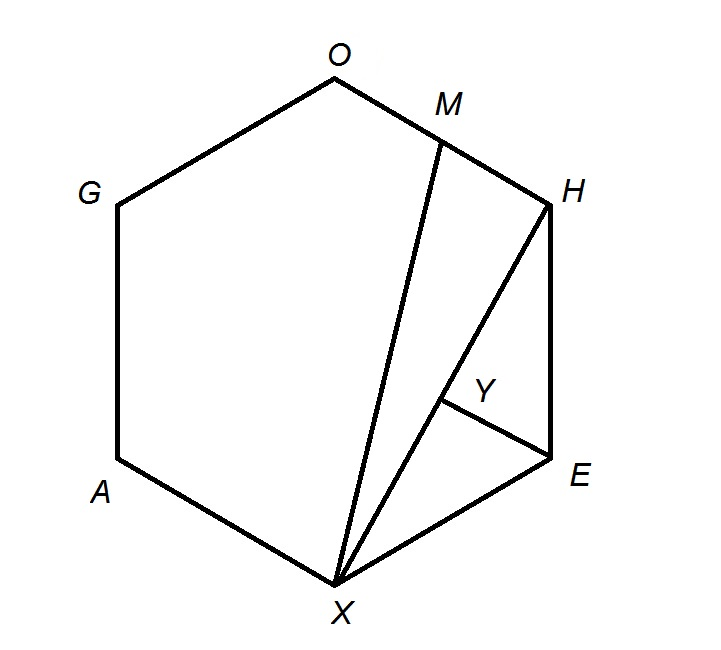Note that the segmentsand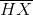have been constructed. Along with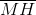, they form right triangle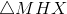with hypotenuse.is the midpoint of, so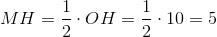.has been divided by drawing the perpendicular from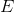to the segment and calling the point of intersection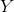.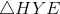is a 30-60-90 triangle with hypotenuse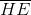, short leg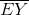, and long leg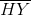, so by the 30-60-90 Triangle Theorem,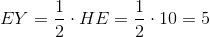and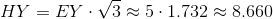For the same reason,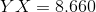, so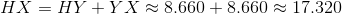By the Pythagorean Theorem,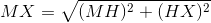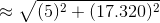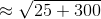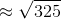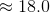when rounded to the nearest tenth.